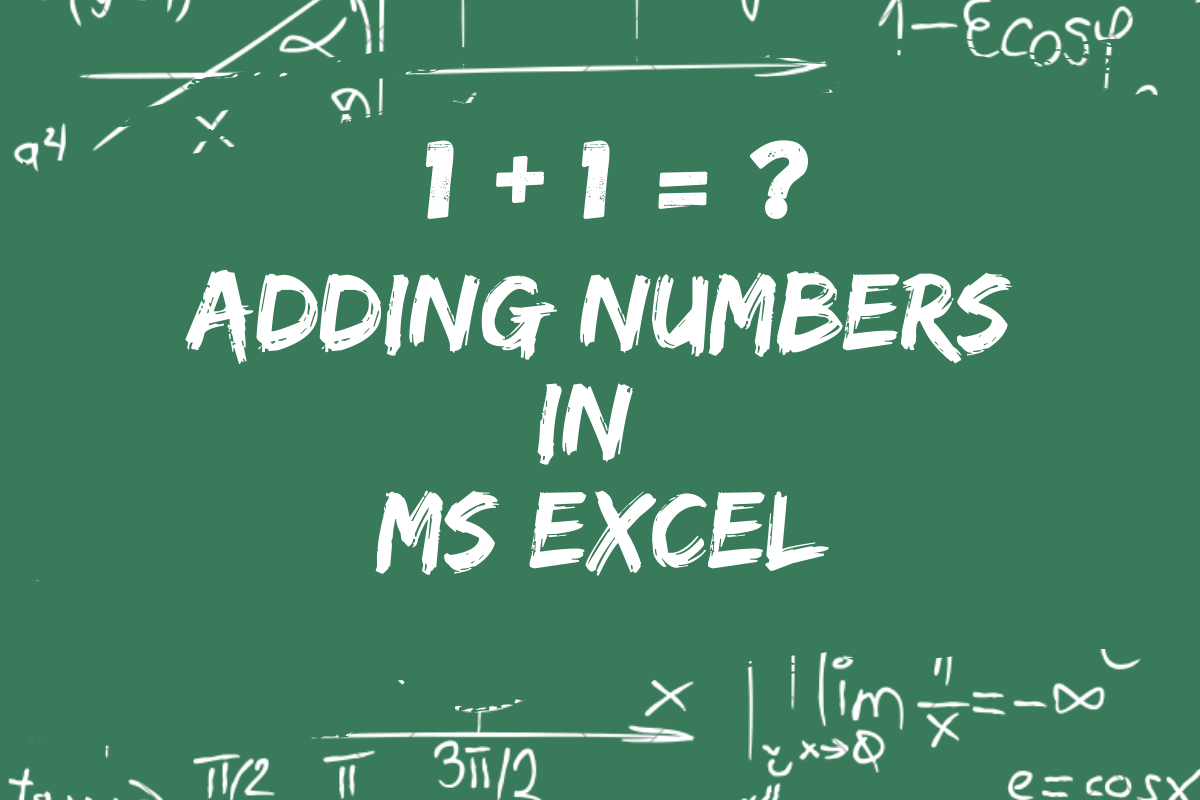# How to Add in Excel? [Easy Guide]Basic arithmetic lays the foundation to get moving further with any complex computations. One of the first arithmetic operations that we were introduced to in school would be Addition. Everyone would be remembering the ‘Two in the mind. One in the finger’ jingle when we were to add the numbers one and two.

In this article, we would be covering the different ways to add numbers using MS Excel and to do that we would be using a list of items purchased on different days to calculate the total quantity bought.

Following are the two techniques that we would be focussing on,

1. The Operand Way
2. The Formula Way

## Method 1 – Adding In Excel Using Operands

Like any formula in MS Excel, we would be beginning with an equal to sign “=” as shown below.

The task here is to add the quantity purchased each day, which would be the cells K3 and L3 in this case. So, we get on with selecting the cell K3 by clicking on it & the cells would turn into something similar to the one shown in the below image.

The operand is the symbol that we would use in MS Excel to carry out a specific operation. For addition, the plus sign (+) is the operand.

Similar to the conventional practice, here too we would be placing the operand in between two variables. So, after selecting the cell K3 type in the plus sign (+) into the cell as shown below.

Once done, click on the next cell L3 which contains the quantity purchased the next day and the cell M3 becomes as shown in the below image.

The long-awaited time is now! Press ENTER and the number resulting from adding the selected cells would appear.

We can’t claim the task to be complete, if we don’t add the quantities for the Chocolates and Biscuits, can we? So, we are just going to do that using a little trick.

We would copy the formula by copying the cell M3 as shown below.

Now select the cells M4 & M5. Press CTRL+V to paste the formula on the selected cells so that we have all the quantities calculated.

## Method 2 – Adding Numbers in Excel Using Formula

Another route to add the required cells using MS Excel is by tapping into its arsenal of in-built formulae. The formula of interest here goes by the term SUM (Self-explanatory, isn’t it?).

Like always, it’s a mandate to start with an equal to “=” sign. The following is the syntax to construct this formula,

=SUM(number1, number2, ……………)

This implies that we can mention either the number or the cell which contains the number we need to add in the place of number1, number2 and so on.

Without any further delay, we shall start with the equal to sign “=” followed by typing the term SUM in cell M3 and open a bracket as shown in the following image.

Once done, we click on K3 containing the quantity of Chips purchased on 01-02-2022 and the contents of the cell M3 gets altered as in the below image.

After this, we would be needing to include a comma “,” following K3 and then click on the next cell L3.